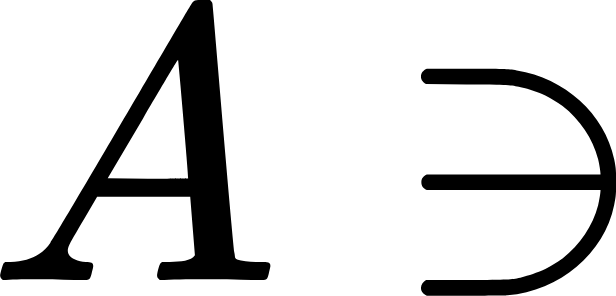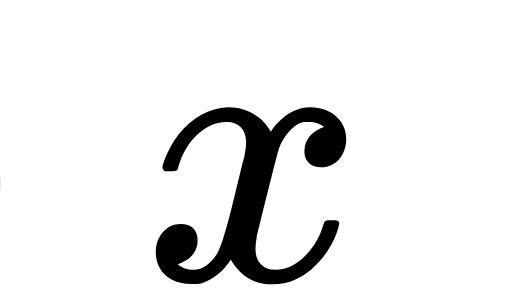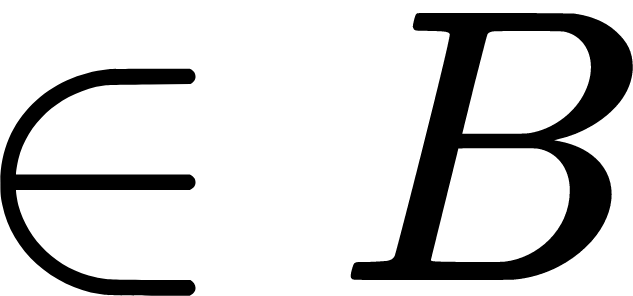# Notation for nonempty intersection

There is no standard mathematical symbol for the intersection relation.

You've probably encountered the symbol for the intersection operation. If $$A$$ and $$B$$ are sets, then "the intersection" of $$A$$ and $$B$$, denoted $$A \cap B$$, is the set of all $$x$$ such that $$x \in A$$ and $$x \in B$$. It's the middle part of a Venn diagram.That's fine, but I'm talking about the intersection relation. We say that $$A$$ "intersects" $$B$$ if there exists some $$x$$ such that $$x \in A$$ and $$x \in B$$. To express the condition using symbols, a common approach is to write $$A \cap B \neq \emptyset$$. It gets the job done, but it's clunky, it's kind of a double negative, and every time I read it, it's like my mind hits a speed bump.

For comparison, consider the equation $$A \cap B = A$$. When I read that equation, I have to do some mental processing before I understand what it is telling me: $$A$$ is a subset of $$B$$. In contrast, when I see the elegant notation $$A \subseteq B$$, the meaning is so clear that I cannot avoid understanding it. (Try reading the word "elephant" and not thinking of an elephant...) I am grateful for the subset symbol. I feel that mathematics would be needlessly hobbled if we were all writing $$A \cap B = A$$ instead of $$A \subseteq B$$.

I propose that we write $$A \ni\!\in B$$ to indicate that $$A$$ intersects $$B$$. The idea is that the condition $$x \in A \cap B$$ can also be written $$A \ni x \in B$$. If you erase the $$x$$ and smush everything together, you get $$A \ni\!\in B$$.Going forward, I plan to write $$A \ni\!\in B$$ in my research papers instead of $$A \cap B \neq \emptyset$$. (I'll always include the definition, and if I have coauthors who would rather not use the new symbol, I won't insist.) In TeX, you can typeset the symbol using the commands \ni\joinrel\in. For disjoint sets, you could use the centernot package to get $$A \centernot {\ni\!\in} B$$, but I'm not sure it's an improvement over the equation $$A \cap B = \emptyset$$.

I'm not the first to suggest notation for intersecting sets. Reddit user nickmcclendon proposed a symbol in 2011. His symbol resembles the amssymb \between symbol, which looks like this: $$A \between B$$. I would be fine with that notation or any other reasonable notation. I just want something to catch on and become standard!